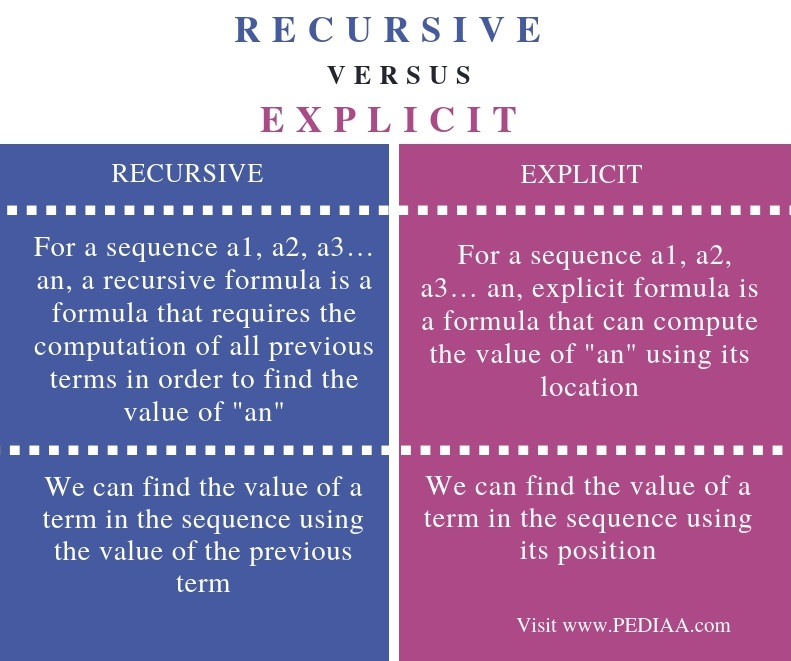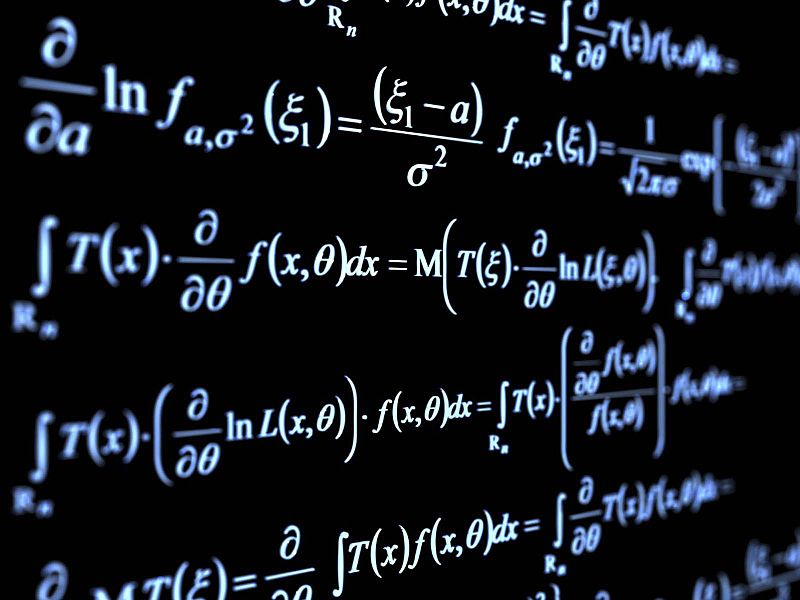# What is the Difference Between Recursive and Explicit

The main difference between recursive and explicit is that a recursive formula gives the value of a specific term based on the previous term while an explicit formula gives the value of a specific term based on the position.

A sequence is an important concept in mathematics. It refers to a set of numbers placed in order. We can represent an arithmetic sequence using a formula. In other words, we can directly compute any term of the sequence using a formula. There are two types of formulas as recursive and explicit formulas. A formula describes a way of finding any term in the sequence.

### Key Areas Covered

1. What is Recursive
– Definition, Functionality
2. What is Explicit
– Definition, Functionality
3. Difference Between Recursive and Explicit
– Comparison of Key Differences

### Key Terms

Explicit Formula, Recursive Formula## What is Recursive

In a recursive formula, we can find the value of a specific term based on the previous term.

For example, assume a formula as follows.

a(n) = a(n-1) +5

The first term of the sequence is a(1)=3

The second term is as follows.

a(2) = a(2-1) + 5

a(2) = a(1) + 5

We can substitute value to the above formula.  Then it will give the result for a(2).

a(2) = 3 + 5

a(2) = 8

Similarly, we can find the third term as follows.

a(3) = a(2) + 5

a(3) = 8+5 = 13

Calculating fourth term is as follows.

a(4) = a(3) + 5

a(4) = 13 + 5 = 18Likewise, we can calculate the values of the terms in the sequence. To find a (4), we need the value of a(3). To find a (3), we need the value of a (2) and to find the value a (2), we need the value of a(1). Therefore, it requires the previous term or terms to find the value of a specific term. That is the functionality of recursive formulas.

## What is Explicit

In explicit formulas, we can find the value of a specific term based on its position.

Assume a formula as follows.

a(n) = 2(n-1) + 4

First term is as follows.

a(1) = 2 (1-1) + 4 = 0 + 4 = 4

Second term is as follows.

a(2) = 2(2-1) + 4 = 2+4 = 6

Third term is as follows.

a(3) = 2(3-1) + 4 = 4 +4 = 8

Fourth term is as follows.

a(4) = 2(4-1) + 4 = 8 + 4 = 12

Likewise, we can find the values of any term in the sequence.

When observing the sequence, it can be seen that it is possible to calculate the value of a specific term using the position. That is how an explicit formula works.

## Difference Between Recursive and Explicit

### Definition

For a sequence a1, a2, a3… an, a recursive formula is a formula that requires the computation of all previous terms in order to find the value of an. For a sequence a1, a2, a3… an, explicit formula is a formula that can compute the value of an using its location. Thus, this is the main difference between recursive and explicit.

### Functionality

In a recursive formula, we can find the value of a term in the sequence using the value of the previous term. However, in an explicit formula, we can find the value of a term in the sequence using its position. Hence, this is another difference between recursive and explicit.

### Conclusion

We can represent a sequence using a formula. A formula can be either recursive or explicit. The main difference between Recursive and Explicit is that Recursive formula gives the value of a specific term based on the previous term while Explicit formula gives the value of a specific term based on the position.

##### Reference:

1.“Recursive Formulas for Arithmetic Sequences.” Khan Academy, Khan Academy, Available here.
2.Mathwords: Removable Discontinuity, Available here.
3.“Explicit Formulas for Arithmetic Sequences.” Khan Academy, Khan Academy, Available here.

##### Image Courtesy:

1.”Random mathematical formulæ illustrating the field of pure mathematics” By Wallpoper (Public Domain) via Commons Wikimedia### About the Author: Lithmee

Lithmee holds a Bachelor of Science degree in Computer Systems Engineering and is reading for her Master’s degree in Computer Science. She is passionate about sharing her knowldge in the areas of programming, data science, and computer systems.